Kubojima, Y., Suzuki, Y., and Tonosaki, M. (2014). "Effect of additional mass on the apparent Young's modulus of a wooden bar by longitudinal vibration," BioRes. 9(3), 5088-5098.

#### Abstract

We investigated the influence of an additional mass bonded on a wooden bar on its apparent Young’s modulus based on a longitudinal vibration theory. Rectangular bars of Sitka spruce (Picea sitchensis Carr.) were used as experimental materials. After bonding an iron piece on a bar, a free-free longitudinal vibration test was performed to obtain the Young’s modulus. Modal analysis was also performed to examine the effect of a knot on the measured Young’s modulus. The Young’s modulus decreased with an increase in mass of iron pieces bonded on the specimen and that in a size of the knot, since the constants required for the frequency equation of longitudinal vibration changed due to the additional mass and the knot. An equation was developed which contains the effects of the mass and position of the iron piece on the constants. The Young’s moduli calculated by this equation resembled the values without an iron piece and the knot. Assuming a knot to be the additional mass, the estimation method used to examine the effect of a knot on the apparent Young’s modulus was proposed. The analysis showed that the higher the resonance mode and the nearer the position to an end, the more effective efforts to reduce the effect of the additional mass will be.

#### Full Article

Effect of Additional Mass on the Apparent Young’s Modulus of a Wooden Bar by Longitudinal Vibration

Yoshitaka Kubojima,a,* Youki Suzuki,a and Mario Tonosaki b

We investigated the influence of an additional mass bonded on a wooden bar on its apparent Young’s modulus based on a longitudinal vibration theory. Rectangular bars of Sitka spruce (Picea sitchensisCarr.) were used as experimental materials. After bonding an iron piece on a bar, a free-free longitudinal vibration test was performed to obtain the Young’s modulus. Modal analysis was also performed to examine the effect of a knot on the measured Young’s modulus. The Young’s modulus decreased with an increase in mass of iron pieces bonded on the specimen and that in a size of the knot, since the constants required for the frequency equation of longitudinal vibration changed due to the additional mass and the knot. An equation was developed which contains the effects of the mass and position of the iron piece on the constants. The Young’s moduli calculated by this equation resembled the values without an iron piece and the knot. Assuming a knot to be the additional mass, the estimation method used to examine the effect of a knot on the apparent Young’s modulus was proposed. The analysis showed that the higher the resonance mode and the nearer the position to an end, the more effective efforts to reduce the effect of the additional mass will be.

Keywords: Additional mass; Free-free condition; Longitudinal vibration test; Knot; Wooden bar; Young’s modulus

Contact information: a: Department of Wood Properties, Forestry and Forest Products Research Institute, 1 Matsunosato, Tsukuba, Ibaraki, 305-8687 Japan; b: Shikoku Research Center, Forestry and Forest Products Research Institute, 2-195 Asakura Nishimachi, Kochi, Kochi, 780-8077 Japan;

* Corresponding author: kubojima@ffpri.affrc.go.jp

INTRODUCTION

Before strength tests are carried out on structural elements and full-size structural timber, the elastic modulus of a specimen must be frequently determined using nondestructive testing methods. Such cases tend to involve vibration tests that are performed by tapping a specimen: generally speaking, a longitudinal vibration test is mainly used as well as a flexural vibration test (Sonoda 2014). Because some structural elements and full-size structural timber contain defects such as knots, their influence on the elastic modulus is important.

To investigate the influence of a defect on flexural vibration test results, an equation of flexural vibration was solved with a Rayleigh method, and the effects of a knot and loss of a section were studied, whereupon a high correlation between the dynamic Young’s modulus and bending strength was obtained (Nakayama 1968; 1974a,b,c; 1975; Nakayama and Aoki 1967; Nakayama and Oshiumi 1970a,b; Nakayama and Yoshikai 1974). In our previous work (Kubojima et al. 2003; 2005; 2006) an equation for the flexural vibration of a beam with additional mass was solved, and the effect of inhomogeneity in a beam on Young’s modulus was investigated.

Consequently, the reason for the inadequacy of the result of the Goens-Hearmon regression method (Goens 1931; Hearmon 1958) based on the Timoshenko bending theory (Timoshenko 1921) was clarified. Yang et al. (2001a,b, 2002) detected defects in wood via nondestructive means; using curvatures of the flexural vibration wave shape measured by modal analysis. Relative frequency shifts in free flexural vibration were investigated, and the defect position was detected (Roohnia et al. 2011). For longitudinal vibration, an inverse solution procedure enabling identification of the defect position from the power spectrum was exploited, and the defect position was effectively identified (Sobue et al.2010).

The present work focused on the inhomogeneity of density (e.g. a knot) in a specimen. Although the frequency equation for the longitudinal vibration of a bar fixed at one end and with a concentrated mass at the other appears in many textbooks (e.g. Timoshenko 1928; Kawai 1953; Kawai 1954), no equation under a free-free condition could be found. The longitudinal vibration test for wood timber is mainly undertaken under a free-free condition. Accordingly, an equation to calculate accurate Young’s modulus values taking into account the additional mass was developed under a free-free condition, whereupon the change in the Young’s modulus caused by the additional mass, and its bonding position on a specimen and the effect of the resonance mode of the longitudinal vibration test were investigated.

THEORY

We assume that an additional mass has no effect on the actual Young’s modulus of wood, even though it may affect the apparent modulus, as obtained by conventional, uncorrected vibrational methods.

The differential equation for the longitudinal vibration of a rectangular bar of width b, thickness h and length l is,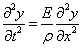(1)

where xytE and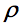are the distance along the bar, the longitudinal displacement, time, Young’s modulus, and density, respectively. Solving Eq. (1), one obtains,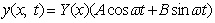(2)

where A and B are constants,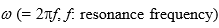is the angular frequency and,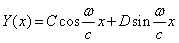(3)

where C and D are constants and c is velocity.

Young’s modulus is expressed by: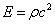(4)

The concentrated mass M is placed at x1 = l and x2 = 0 (Fig. 1). Since the axial force does not exist at each extremity,(5)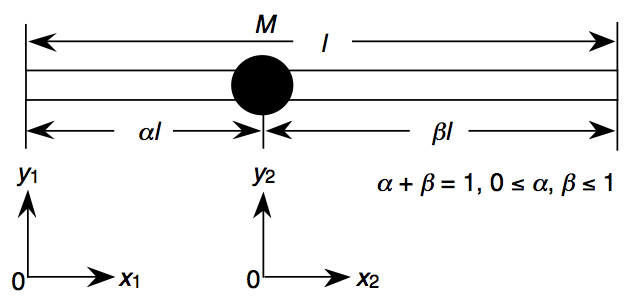Fig. 1. A beam with additional mass

Since both parts of the bar are connected and the difference in the axial force in the bar is equal to the inertia force exerted by the concentrated mass at x1 = l and x2 = 0,(6)

where, S is the cross sectional area of the bar.

From Eqs. 2, 3, 5, and 6,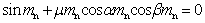(7)

where,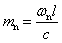(8)

and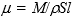is the ratio of the concentrated mass to that of the bar. Suffix n is the resonance mode number. Equation 7 is the frequency equation for the free-free longitudinal vibration with the concentrated mass.

i) If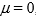, then Eq. 7 is as follows: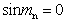(9)

This is the frequency equation for a bar that has free ends without the concentrated mass.

ii) If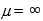, then Eq. 7 is as follows: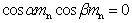(10)

If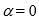or 1, then Eq. (10) is as follows: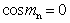(11)

This is the frequency equation for a bar under a fixed-free condition without the concentrated mass.

EXPERIMENTAL

Specimens

Sitka spruce (Picea sitchensis Carr.) was used for the specimens, which were 300 mm (longitudinal, L) long, 30 mm (radial, R) wide, and 5 mm (tangential, T) thick.

The specimens were conditioned at 20 °C and 65 % relative humidity until the weight was constant. The tests were conducted under the same conditions.

Longitudinal Vibration Test

To obtain the Young’s modulus, free-free longitudinal vibration tests were conducted by the following procedure: The test bar was placed on a small sponge at the position of x = l/2. The vibration was excited in the longitudinal direction at one end by a hammer, while the motion of the first mode of the bar was detected by a microphone at the other end. The signal was processed through an FFT digital signal analyzer to yield high-resolution resonance frequencies. The sampling rate of the microphone signal and the FFT resolution were 20 to 12500 Hz and 0.25 Hz, respectively. A diagram of the experimental setup is shown in Fig. 2. The vibration test was conducted for the specimen without and with iron pieces 1 (0.63 g,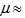0.033), 2 (1.28 g,0.067), and 3 (1.91 g,0.099). The iron pieces were bonded at x = 0, 0.1l, 0.2l, 0.3l, 0.4l, and 0.5l on the LR-plane with two-sided adhesive tape, which meant the same specimen could be used for bonding an iron piece on 6 positions of the specimen.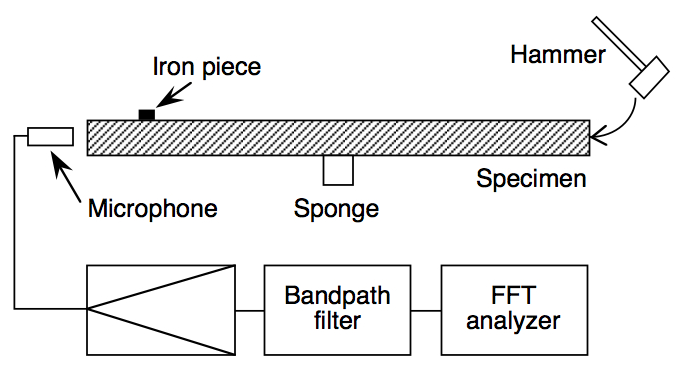Fig. 2. A diagram of the experimental setup

Modal analysis

To simulate the resonance frequency in the L-direction of a specimen with a knot, modal analysis was conducted using an existing finite element method program, “ANSYS 14.5” developed by ANSYS, Inc., which is a library program of Agriculture, Forestry and Fisheries Research Information Technology Center (AFFRIT), Ministry of Agriculture, Forestry and Fisheries (MAFF), Japan. The resonance frequency of the first mode was calculated with the block Lanczos method. The dimensions of the specimen were 300 mm (L) long, 30 mm (T) wide, and 5 mm (R) thick. Figure 3 shows the finite element mesh of the specimen. This model consisted of two dimensional quadrangle elements. The parameters used for the modal analysis were as follows: density was 330 kg/cm3 and Young’s modulus in the L-, T-, and R-directions were 7.35, 0.29, and 0.59 GPa, respectively, the shear moduli of the LT-, RT-, and LR-planes were 0.343, 0.015, and 0.637 GPa, respectively, and Poisson’s ratio of the LT-plane was 0.60. They were values of the normal part of Japanese cedar (Cryptomeria japonicaD. Don) (Forestry and Forest Products Research Institute 2004). The density of the knot was 900 kg/cm3 (Nakamura 1972; Nakayama and Yoshiaki 1974). The positions of the knot were x = 0.1l, 0.2l, 0.3l, 0.4l, and 0.5l (l = 300 mm). The diameters of the knot were 10, 20, and 29 mm.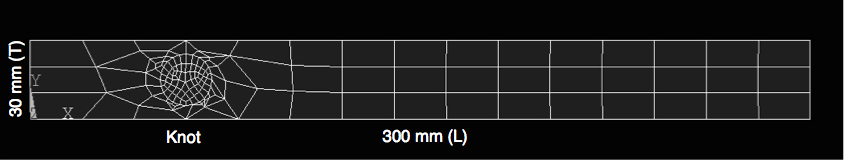Fig. 3. Finite element model of a specimen with a knot

RESULTS AND DISCUSSION

Figure 4 shows the results of the longitudinal vibration test. The Young’s modulus calculated normally, namely, from Eqs. 4, 8, and 9, declined with the increase in additional mass.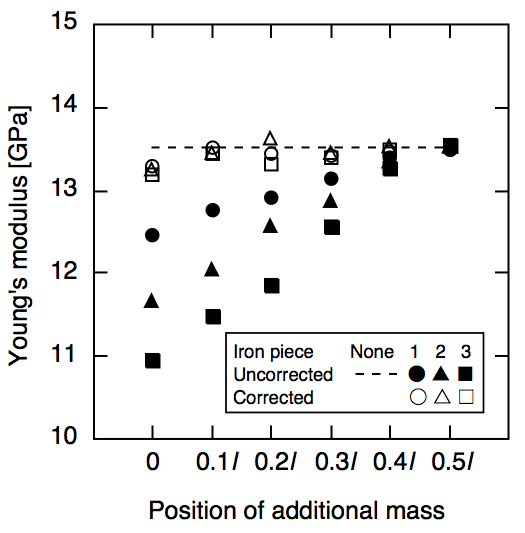Fig. 4. Uncorrected Young’s modulus, and Young’s modulus corrected by the mass of the iron pieces using Eq. 7

Conversely, given various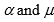, Eq. 7 was solved by the “Mathematica Ver. 9” software (Wolfram Research Co., Ltd.), which is a library program of AFFRIT, MAFF, Japan; the obtained mn was substituted into Eq. 8, and Young’s modulus was calculated from Eq. 4. The Young’s modulus values obtained in such manner were almost equivalent to that without the additional mass, which meant the effect of the additional mass on the Young’s modulus could be corrected using Eq. 7.

Figure 5 shows the results of the modal analysis. The Young’s modulus calculated normally from Eqs. 4, 8, and 9, declined with the increase in the diameter of the knot. Here, a rectangular bar of width b, thickness h, length l and a circular knot of radius r is considered (Fig. 6). The densities of the knot and other part are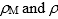, respectively.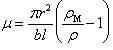(12)

When the diameters of the knot are 10, 20, and 29 mm, values of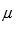are 0.015, 0.060, and 0.13, respectively.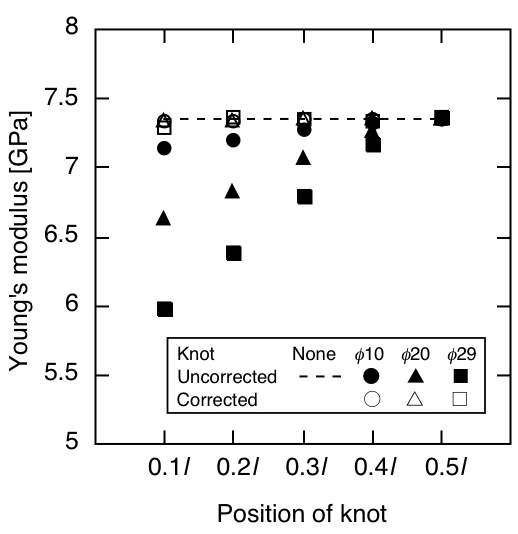Fig. 5. Uncorrected Young’s modulus, and Young’s modulus corrected by the size of the knot using Eq. 7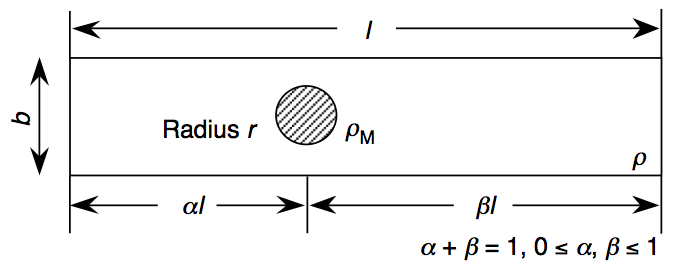Fig. 6. A rectangular beam with a circular knot

Given various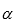and, Eq. 7 was solved by the “Mathematica Ver. 9” software; the obtained mn was substituted into Eq. 8, and Young’s modulus was calculated from Eq. 4. The Young’s modulus values obtained in such manner were almost equivalent to that without the knot, which meant the effect of the knot on the Young’s modulus could be corrected using Eq. 7.

Next, a knot is assumed to be the additional mass. Although the Young’s modulus of wood itself is not changed by the additional mass, the value calculated by Eq. 9 is lower than the accurate value. For example, we consider that Young’s modulus is apparently reduced by a knot by 10 % if Eq. (9) is used. The first resonance mode is used.

Young’s modulus of the n-th resonance mode using Eq. 9 is as follows: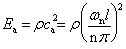(13)

Young’s modulus of the n-th resonance mode using Eq. 7 is as follows: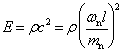(14)

When n = 1 and Ea = 0.9E, from Eqs. 13 and 14,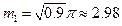.

Substituting variousvalues and m1 = 2.98 into Eq. 7,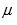is calculated and r obtained from Eq. 12.

The shaded part of Fig. 7 shows the region of 0.9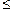Ea/E1. Japanese cedar (Cryptomeria japonicaD. Don) is considered and bl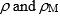values are 30 mm (T), 300 mm (L), 330 kg/m3, and 900 kg/m3, respectively.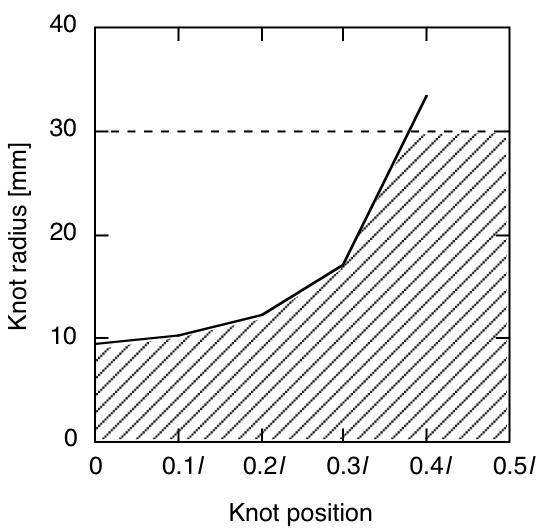Fig. 7. Relationship between the knot radius and the knot position when the decrease in the Young’s modulus is not more than 10 %

The higher resonance mode of longitudinal vibration is less influenced by the specimen setting conditions of a specimen (Arima et al. 1990; Aratake et al. 1992). The additional mass relates to the specimen setting conditions as shown in the theory section. Thus, the effect of the resonance mode was investigated. The additional mass is placed at the anti-nodal positions of the free-free longitudinal vibration shown in Table 1.

Table 1. Nodal and Anti-Nodal Positions of Free-Free Longitudinal Vibration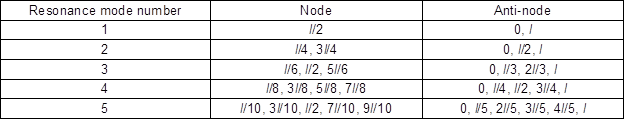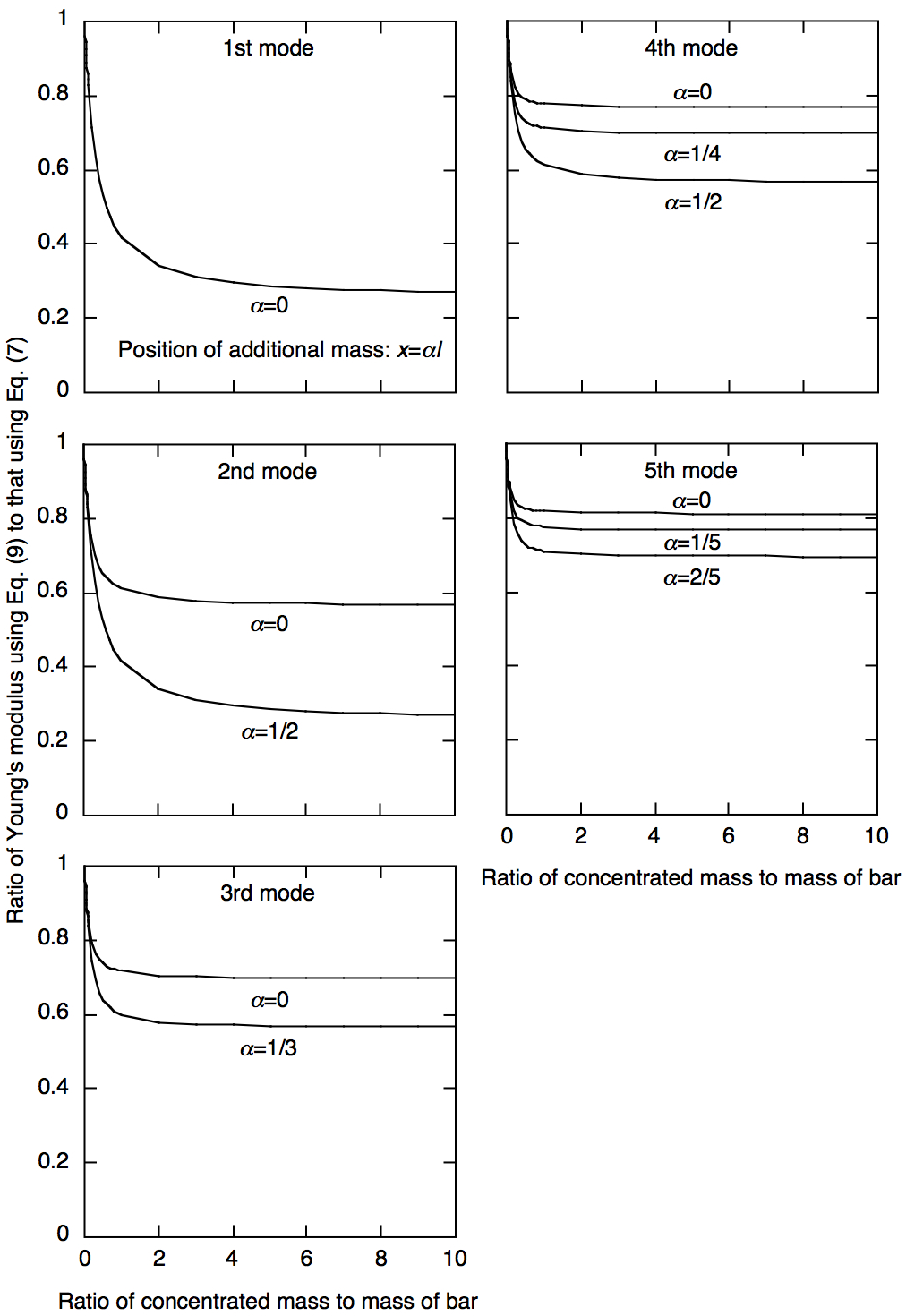Fig. 8. The effect of the resonance mode on the decrease in Young’s modulus by the additional mass

The ratio of Young’s modulus using Eq. (9) to that using Eq. (7) is expressed based on Eqs. 13 and 14 as follows: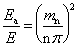(15)

Figure 8 shows the relationship between Ea/E and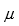for n = 1 to 5. The value of Ea/E decreased with increases inandwhile it decreased less with an increase in n. The longitudinal displacement of vibration decreases with an increase in n, which means that ensuring a higher resonance mode and a position nearer to the end among the anti-nodal positions are effective means of reducing the effect of the additional mass. Hence, when the longitudinal vibration is used, as high a resonance mode as possible should be used.

CONCLUSIONS

1. The effects of the additional mass and the knot on the apparent Young’s modulus could be corrected using Eq. 7.
2. Assuming a knot to be the additional mass, a method of estimation to examine the effect of a knot on the apparent Young’s modulus was proposed.
3. Ensuring a higher resonance mode and a position nearer to an end among the anti-nodal positions will be effective in reducing the effect of the additional mass.

ACKNOWLEDGMENTS

We would especially like to thank Prof. Akio Koizumi of Hokkaido University for his suggestions in conducting our work. This work was financially supported by the Research grant #201402 of the Forestry and Forest Products Research Institute.

REFERENCES CITED

Aratake, S., Arima, T., Sakoda, T., and Nakamura, Y. (1992). “Estimation of modulus of rupture (MOR) and modulus of elasticity (MOE) of lumber using higher natural frequency of log in pile of logs. Possibility of application for sugi scaffolding board,” Mokuzai Gakkaishi 38(11), 995-1001.

Arima, T., Hayamura, S., Maruyama, N., Miyazawa, S., and Furusawa, S. (1990). “Evaluation for modulus of elasticity and weight change of lumber by sound analysis,” J. Soc. Mater. Sci. Jpn.39(444), 1228-1234.

Forestry and Forest Products Research Institute (2004). Wood Industry Handbook, Maruzen, Tokyo.

Goens, E. (1931). “Über die Bestimmung des Elastizitätsmodulus von Stäben mit Hilfe von Biegungsschwingungen,” Annal. Phys. 5F. 11(6), 649-678.

Hearmon, R. F. S. (1958) “The influence of shear and rotatory inertia on the free flexural vibration of wooden beams,” Brit. J. Appl. Phys. 9(10), 381-388.

Kawai, R. (1953). “A method of correcting the effect of the mass of a bar with a concentrated mass at one end in longitudinal fundamental vibration,” Trans. Jpn. Soc. Mech. Eng. 19(86), 112-114.

Kawai, R. (1954). “Higher modes of the longitudinal vibration of a bar fixed at one end and carrying a concentrated mass at other,” Trans. Jpn. Soc. Mech. Eng. 20(95), 508-509.

Kubojima, Y., Tonosaki, M., and Yoshihara, H. (2003). “Effect of the additional mass applied at an end of a wooden beam on its flexural vibrational properties,” Wood Ind. 58(8), 370-372.

Kubojima, Y., Tonosaki, M., and Yoshihara, H. (2005). “Effect of additional mass on Young’s modulus of a wooden beam,” J. Test. Eval. 33(4), 278-282.

Kubojima, Y., Yoshihara, H., and Tonosaki, M. (2006). “Young’s modulus of a wooden beam with inhomogeneity of density obtained by flexural vibration test,” J. Wood. Sci. 52(1), 20-24.

Nakamura, Y. (1972). “Studies on stress grading for structural laminae taken from woods in southern Kyushu,” Bull. Miyazaki Univ. For. 6, 1-82.

Nakayama, Y. (1968). “Studies on the dynamic Young’s modulus of wooden beam (I) The dynamic Young’s modulus and the bending strength,” Bull. Kochi Univ. For. 2, 17-32.

Nakayama, Y. (1974a). “On reduction of dynamic Young’s modulus by reduced section in wooden beam,” Res. Rep. Kochi Univ. Agr. Sci. 23(12), 115-130.

Nakayama, Y. (1974b). “Fundamental resonant frequency of wooden beam containing the decrease section,” Mem. Fac. Agr. Kochi Univ. 26, 1-59.

Nakayama, Y. (1974c). “Vibrational property of wooden beam containing the decrease section. On the perpendicular bending moment to the decreased plain,” Mokuzai Gakkaishi 20(1), 1-8.

Nakayama, Y. (1975). “Non-destructive test of wooden beam by vibrational method. Estimation of modulus of rupture in bending of beam containing an artificial circular hole,” Mokuzai Gakkaishi21(7), 402-409.

Nakayama, Y., and Aoki, A. (1967). “Studies on the dynamic elasticity of wood. Part I. The measuring apparatus and measuring methods of vibration of wood,” Res. Rep. Kochi Univ. Natural Sci. II 16, 167-176.

Nakayama, Y., and Oshiumi, S. (1970a). “Studies on the dynamic elasticity of wood. Part II. Effect of the artificial round hole and the artificial round knot,” Res. Rep. Kochi Univ. Agr. Sci. 19, 125-135.

Nakayama, Y., and Oshiumi, S. (1970b). “Studies on the dynamic elasticity of wood. Part III. Effect on the loss of the section in cantilever wooden beam,” Res. Rep. Kochi Univ. Agr. Sci. 19, 137-145.

Nakayama, Y., and Yoshikai, Y. (1974). “Estimation of modulus of rupture in bending of sugi beam with knots by vibrational method,” Res. Rep. Kochi Univ. Agr. Sci. 23(16), 155-166.

Roohnia, M., Manouchehri, N., Tajdini, A., Yaghmaeipour, A., and Bayramzadeh, V. (2011). “Modal frequencies to estimate the defect position in a flexural wooden beam,” BioResources 6(4), 3676-3686.

Sobue, N., Fujita, M., Nakano, A., and Suzuki, T. (2010). “Identification of defect position in a wooden beam from the power spectrum of longitudinal vibration,” J. Wood Sci. 56(2), 112-117.

Sonoda, S. (2014). “Acoustic vibrations and their higher harmonics for non-destructive measurement techniques,” Wood Ind. 69(4), 183-186.

Timoshenko, S. P. (1921). “On the correction for shear of the differential equation for transverse vibrations of prismatic bars,” Phil. Mag. 6 Ser. 41, 744-746.

Timoshenko, S. P. (1928). Vibration Problems in Engineering, D. Van Nostrand Company Inc, New York.

Yang, X., Ishimaru, Y., Iida, I., and Urakami, H. (2001a). “Application of modal analysis by transfer function to nondestructive testing of wood. I. Determination of localized defects in wood by the shape of the flexural vibration wave,” Mokuzai Gakkaishi 47(4), 304-310.

Yang, X., Amano, T., Ishimaru, Y., Iida, I., and Urakami, H. (2001b). “Application of modal analysis by transfer function to nondestructive testing of wood. II. Modulus of elasticity evaluation of sections of differing quality within a wooden beam by the curvature of the flexural vibration wave,” Mokuzai Gakkaishi 47(5), 412-419.

Yang, X., Ishimaru, Y., Iida, I., and Urakami, H. (2002). “Application of modal analysis by transfer function to nondestructive testing of wood. III. Determination of knots and estimation of elastic modulus distribution in wood by the curvature ratio of the flexural vibration wave shape,” Mokuzai Gakkaishi 48(1), 16-22.

Article submitted: May 1, 2014; Peer review completed: July 2, 2014; Revised version received and accepted: July 2, 2014; Published: July 10, 2014.